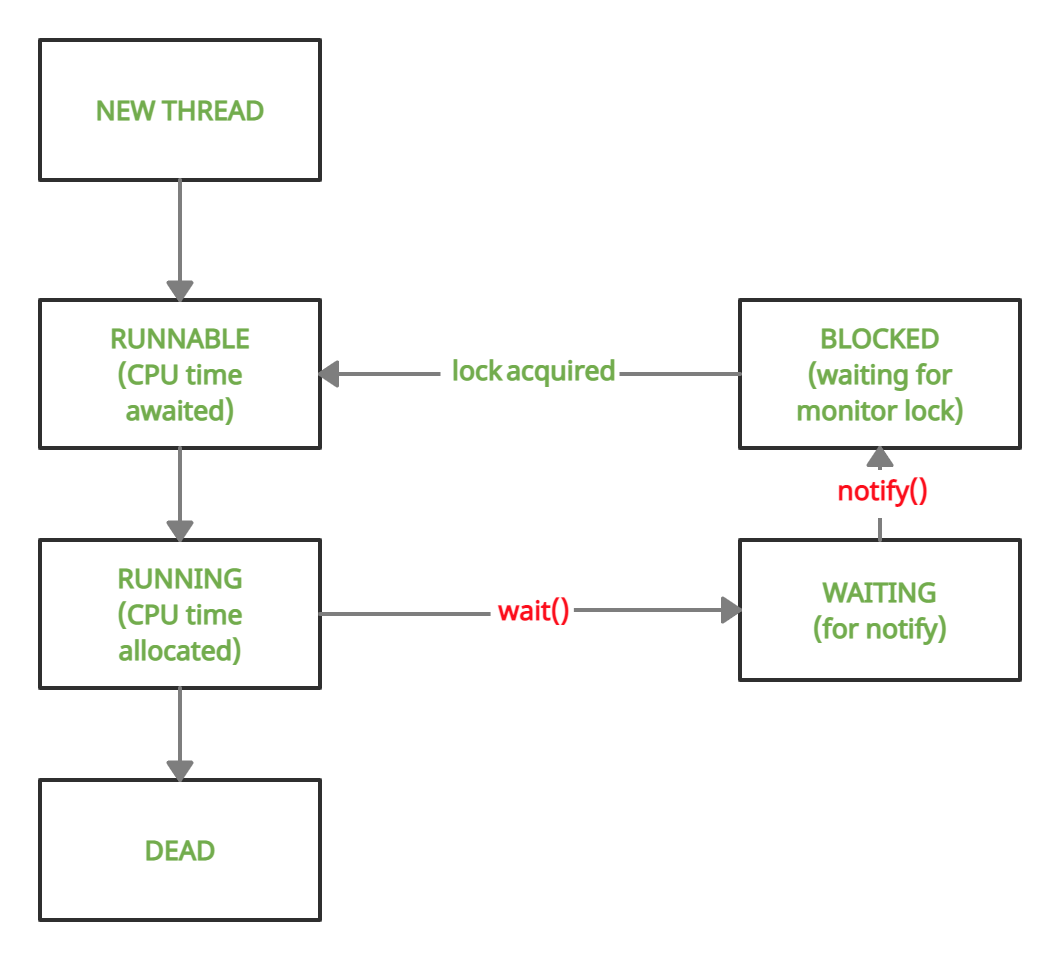Related Articles

# Difference Between wait() and notify() in Java

• Last Updated : 09 Sep, 2021

wait() and notify() are methods of the Object class. They were introduced to part ways with polling, which is the process of repeatedly checking for a condition to be fulfilled. Polling wastes CPU resources considerably, hence it is not preferred.

wait() has 3 variations:

Attention reader! Don’t stop learning now. Get hold of all the important Java Foundation and Collections concepts with the Fundamentals of Java and Java Collections Course at a student-friendly price and become industry ready. To complete your preparation from learning a language to DS Algo and many more,  please refer Complete Interview Preparation Course.

1. The basic version which does not take any argument

```public final void wait()
// will cause thread to wait till notify is called```

2. The version taking a single timeout argument

```public final void wait(long timeout)
// will cause thread to wait either till notify is called or
// till timeout (One which occurs earlier)```

3. The version taking a timeout argument as well as a nanosecond argument for extra precision

```public final void wait(long timeout,
int nanoseconds)```

notify() has 1 signature

```public final void notify()
// Function does not take any argument```Below is demonstration of wait() and notify() method:

## Java

 `// Java Program to demonstrate usage of wait() and notify()` `class` `demo {``    ``// variable to check if part1 has returned``    ``// volatile used to prevent threads from``    ``// storing local copies of variable``    ``volatile` `boolean` `part1done = ``false``;` `    ``// method synchronized on this``    ``// i.e. current object of demo``    ``synchronized` `void` `part1()``    ``{``        ``System.out.println(``"Welcome to India"``);``        ``part1done = ``true``;``        ``System.out.println(``            ``"Thread t1 about to surrender lock"``);``        ``// notify the waiting thread, if any``        ``notify();``    ``}` `    ``// method synchronized on this``    ``// i.e. current object of demo``    ``synchronized` `void` `part2()``    ``{``        ``// loop to prevent spurious wake-up``        ``while` `(!part1done) {``            ``try` `{``                ``System.out.println(``"Thread t2 waiting"``);``                ``// wait till notify is called``                ``wait();``                ``System.out.println(``                    ``"Thread t2 running again"``);``            ``}``            ``catch` `(Exception e) {``                ``System.out.println(e.getClass());``            ``}``        ``}``        ``System.out.println(``"Do visit Taj Mahal"``);``    ``}``}` `public` `class` `Main {` `    ``public` `static` `void` `main(String[] args)``    ``{` `        ``// Make an instance of demo calss``        ``demo obj = ``new` `demo();` `        ``// Thread t1 will call part1()``        ``Thread t1 = ``new` `Thread(``new` `Runnable() {``            ``public` `void` `run() { obj.part1(); }``        ``});` `        ``// Thread t2 will call part2()``        ``Thread t2 = ``new` `Thread(``new` `Runnable() {``            ``public` `void` `run() { obj.part2(); }``        ``});` `        ``// Start t2 and then t1``        ``t2.start();``        ``t1.start();``    ``}``}`
Output
```Thread t2 waiting
Welcome to India
Do visit Taj Mahal```

Various Exception

A. wait()

• It is mandatory to enclose wait() in a try-catch block because if a thread present in the waiting state gets interrupted, then it will throw InterruptedException.
• The other two variations of wait housing parameters will throw IllegalArgumentException if the value of timeout is negative or the value of nanoseconds is not in the range 0 to 9,99,999.

Below is the implementation for the exception handling.

## Java

 `// Program demonstrating occurrence of InterruptedException` `class` `demo {``    ``volatile` `boolean` `part1done = ``false``;` `    ``synchronized` `void` `part1()``    ``{``        ``System.out.println(``"Welcome to India"``);``        ``part1done = ``true``;``        ``// notify() has been commented, waiting``        ``// thread remains waiting forever notify();``    ``}` `    ``synchronized` `void` `part2()``    ``{``        ``while` `(!part1done) {``            ``try` `{``                ``wait();``            ``}``            ``catch` `(Exception e) {``                ``System.out.println(``"Exception : "``                                   ``+ e.getClass());``                ``// quit program after exception is thrown``                ``System.exit(-``1``);``            ``}``        ``}``        ``System.out.println(``"Do visit Taj Mahal"``);``    ``}``}` `public` `class` `Main {` `    ``public` `static` `void` `main(String[] args)``    ``{``        ``// Make an instance of demo class``        ``demo obj = ``new` `demo();` `        ``// Thread t1 will call part1()``        ``Thread t1 = ``new` `Thread(``new` `Runnable() {``            ``public` `void` `run() { obj.part1(); }``        ``});` `        ``// Thread t2 will call part2()``        ``Thread t2 = ``new` `Thread(``new` `Runnable() {``            ``public` `void` `run() { obj.part2(); }``        ``});` `        ``// Start t2 and then t1``        ``t2.start();``        ``t1.start();` `        ``// This is a counter which will``        ``// interrupt Thread t2 after 3 seconds``        ``long` `startTime = System.currentTimeMillis();``        ``while` `(``true``) {``            ``if` `(System.currentTimeMillis() - startTime``                ``> ``3000``)``                ``t2.interrupt();``        ``}``    ``}``}`
Output
```Welcome to India
Exception : class java.lang.InterruptedException```

B. notify()

Unlike wait(), the notify method does not throw an InterruptedException hence it is not mandatory to house it inside a try-catch block

Note:

• wait() and notify() both have a tendency to throw IllegalMonitorStateException
• This occurs when a thread is holding the monitor lock of object A and tries to call wait or notify on object B.
• In all the preceding examples, the methods were synchronized on “this” i.e. the object used to call those methods (obj). Also, the wait() & notify() were being called as this.wait() and this.notify() (usage of this was redundant). Hence, there was no issue.
• In the below example, the methods part1 and part2 are now synchronized on an Integer object, but wait() & notify() are still being called on the object which called these methods (obj).
• This causes an IllegalMonitorStateException

Below is the implementation of the exception handling.

## Java

 `// Program to demonstrate IllegalMonitorStateException` `class` `demo {``    ``volatile` `boolean` `part1done = ``false``;``    ``// Made an Integer object a``    ``// and set it randomly to 5``    ``Integer a = ``5``;` `    ``void` `part1()``    ``{``        ``// Synchronized code on a``        ``synchronized` `(a)``        ``{``            ``System.out.println(``"Welcome to India"``);``            ``part1done = ``true``;``            ``// calling this.notify()``            ``notify();``        ``}``    ``}` `    ``void` `part2()``    ``{``        ``// Synchronized code on a``        ``synchronized` `(a)``        ``{``            ``while` `(!part1done) {``                ``try` `{``                    ``// calling this.wait()``                    ``wait();``                ``}``                ``catch` `(Exception e) {``                    ``System.out.println(``"Exception: "``+e.getClass());``                    ``System.exit(-``1``);``                ``}``            ``}``            ``System.out.println(``"Do visit Taj Mahal"``);``        ``}``    ``}``}` `public` `class` `Main {` `    ``public` `static` `void` `main(String[] args)``    ``{` `        ``// Make an instance of demo class``        ``demo obj = ``new` `demo();` `        ``// Thread t1 will call part1()``        ``Thread t1 = ``new` `Thread(``new` `Runnable() {``            ``public` `void` `run() { obj.part1(); }``        ``});` `        ``// Thread t2 will call part2()``        ``Thread t2 = ``new` `Thread(``new` `Runnable() {``            ``public` `void` `run() { obj.part2(); }``        ``});` `        ``// Start t2 and then t1``        ``t2.start();``        ``t1.start();``    ``}``}`
Output
`Exception: class java.lang.IllegalMonitorStateException`

To fix the above code, just replace notify() with a.notify() on line 19 and wait() with a.wait() on line 31.

My Personal Notes arrow_drop_up